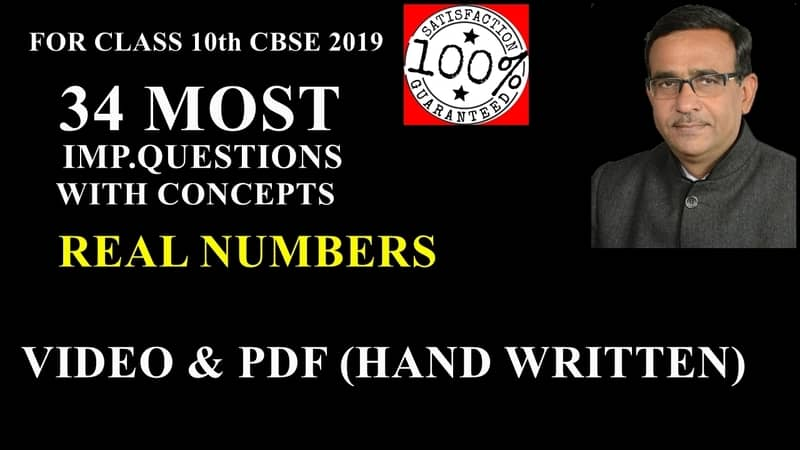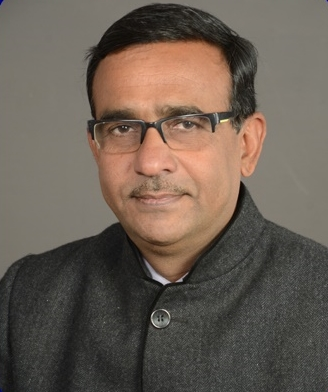## by R B Gautam

3544 Users Enrolled
Class 10 , Maths
Board: CBSE & State Boards , Language: Hinglish

Mode- Online
Contains- 34 Videos
Access Duration- Till 31 Mar 2020

## WHAT WILL I LEARN?

Real Numbers

* After Solving these 34 Questions of real numbers, you can solve all questions of this topic in board examination

## Course Description

Real Numbers

* 34 Most Important Questions with Concepts

* Solution of each question  in Video and PDF (hand written) format

## Curriculum

10th Maths Ch.1 Real Numbers

• Concept of Euclid’s Division Lemma and algorithm Preview Concept of Euclid’s Division Lemma and algorithm Preview
• Q1.Using Euclid’s algorithm, find the HCF of 240 and 228.
• Q3. Using Euclid’s division algorithm finds the HCF of 1656 and 4025.
• Q4.Find the HCF by Euclid’s division algorithm of the numbers 92690, 7378 and 7161. Preview Q4.Find the HCF by Euclid’s division algorithm of the numbers 92690, 7378 and 7161. Preview
• Q5.Obtain the HCF of 420 and 272 by using Euclid’s division algorithm and verify the same by using fundamental theorem of arithmetic.
• Q6.Find the HCF of 180, 252 and 324 using Euclid’s division lemma.
• Q7.Find the greatest number that will divide 445, 572 and 699 leaving remainders 4,5 and 6 respectively .
• Q8.Find HCF of 90 and 126 by Euclid’s division algorithm. Also, find their LCM and verify that LCM × HCF = Product of two numbers.
• Q9.If d is the HCF of 45 and 27, find x and y , satisfying d = 45 x + 27 y .
• Q10.If d is the HCF of 56 and 72, find x and y satisfying d = 56 x + 72 y .
• Q11.Find HCF of 81 and 237 and express it as a linear combination of 81 and 237 i.e. , HCF (81, 237) = 81x+237 y for some x and y.
• Q12.Show that one and only one out of n, n+2 or n + 4 is divisible by 3 , where n is any positive integers .
• Q13.Write whether the square of any positive integer can be of the form 3m + 2 , where m is a natural number. Justify your answer.
• Q14.Show that the square of any positive integer is of the form 4m or 4m + 1, where m is any integer.
• Q15.Use Euclid division lemma to show that the square of any positive integer cannot be of the form 5m+ 2 or 5m + 3 for integer m.
• Q16.Use Euclid’s division lemma to show that the cube of any positive integer is either of the form 9m or 9m + 1 or 9m + 8.
• Q17.Show that any positive odd integer is of the form 6q+1 or 6q+3 or 6q+5, where q is some integer.
• Q18.Prove that n2 – n is divisible by 2 for every positive integer n.
• Q19.For any positive integer n, prove that n3 – n is divisible by 6.
• Q20. In a school, the duration of a period in junior sections is 40 minutes and senior section is 1 hour. If the first bell for each section rings at 9: 00 a. m, when will the two bells ring together again?
• Q21.Two tankers contain 620 liters and 840 liters of diesel respectively. Find the maximum capacity of a container which can measure the diesel of both the tankers in exact number of times.
• Q22.Concept of Fundamental Theorem of Arithmetic.
• Q23.Find the missing number in the following factorization.
• Q24.Complete the following factor tree and find the composite number x and y.
• Q25.Find the smallest natural number by which 1200 should be multiplied so that the square root of the product is a rational number.
• Q26.Check whether (i) (15 )n (ii) (4 )n (iii) ( 30 )n can end with the digit zero.
• Q27.Explain whether 3 × 12 × 101+4 is a prime number or a composite number.
• Q28.Write the HCF and LCM of the smallest odd composite number and the smallest odd prime number. If an odd number p divides q 2, then will it divide q3, also? Explain.
• Q29.If p is a prime number then prove that √p is irrational.
• Q30.Prove that √(P )+ √q is irrational, where p and q are primes.
• Q31.Prove that √5 is an irrational number and hence show that 2- √5 is also an irrational number.
• Q32.Is (π- 22/7) a rational number, an irrational number or zero?
• Q33.Can two numbers have 15 as their HCF and 175 as their LCM? Give reasons.
• Q34.The LCM of two numbers is 14 times their HCF . The sum of LCM and HCF is 600. If one number is 280, then find the other number .### R B Gautam

Mathematics | Class 9th to 12th | 25+ Years Experience | R B Gautam Sir is a well-known for his expertise in Math’s. He is serving the society by providing students with quality content to achieve 100 out of 100 in their math’s exams. R B Gautam Sir helps students by solving and explaining major problems faced by students in their exams.

## 299 99

Includes 18 % GST

Mode- Online

Contains- 34 Videos

Access Duration- Till 31 Mar 2020